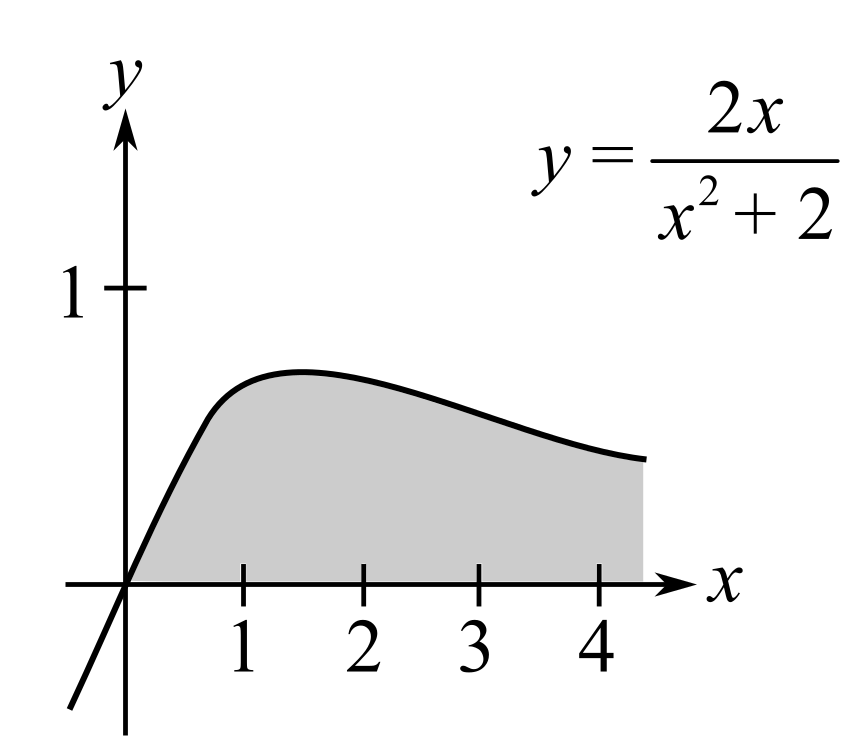Chapter 6, Problem 58RE### Calculus: An Applied Approach (Min...

10th Edition
Ron Larson
ISBN: 9781305860919

#### Solutions

Chapter
Section### Calculus: An Applied Approach (Min...

10th Edition
Ron Larson
ISBN: 9781305860919
Textbook Problem
1 views

# Area of a Region In Exercises 57–60, find the area of the unbounded shaded region. y = 2 x x 2 + 2To determine

To calculate: The area under the shaded region under the graph of the function y=2xx2+2.Explanation

Given Information:

The equation, y=2xx2+2

And the graph is,

Formula used:

From definition of improper integral.

If on the interval [a,), the function is continuous, then

af(x)dx=limbabf(x)dx

The integral formula:

1xdx=|x|+C

Calculation:

Consider the provided equation:

02xx2+2dx

And the graph,

Use the definition of improper integral af(x)dx=limbabf(x)dx

And simplify as

02xx2+2dx=limb02xx2+2dx

In the provided integral,

Assume x2+2=u

Now, differentiate

2xx2

### Still sussing out bartleby?

Check out a sample textbook solution.

See a sample solution

#### The Solution to Your Study Problems

Bartleby provides explanations to thousands of textbook problems written by our experts, many with advanced degrees!

Get Started

#### In Exercises 21-24, find the distance between the given points. 22. (1, 0) and (4, 4)

Applied Calculus for the Managerial, Life, and Social Sciences: A Brief Approach

#### In problems 15-22, simplify by combining like terms. 21.

Mathematical Applications for the Management, Life, and Social Sciences

#### Which number is the best choice for the value of a in the following graph? a) e b) 1 c) 2 d) 3

Study Guide for Stewart's Single Variable Calculus: Early Transcendentals, 8th